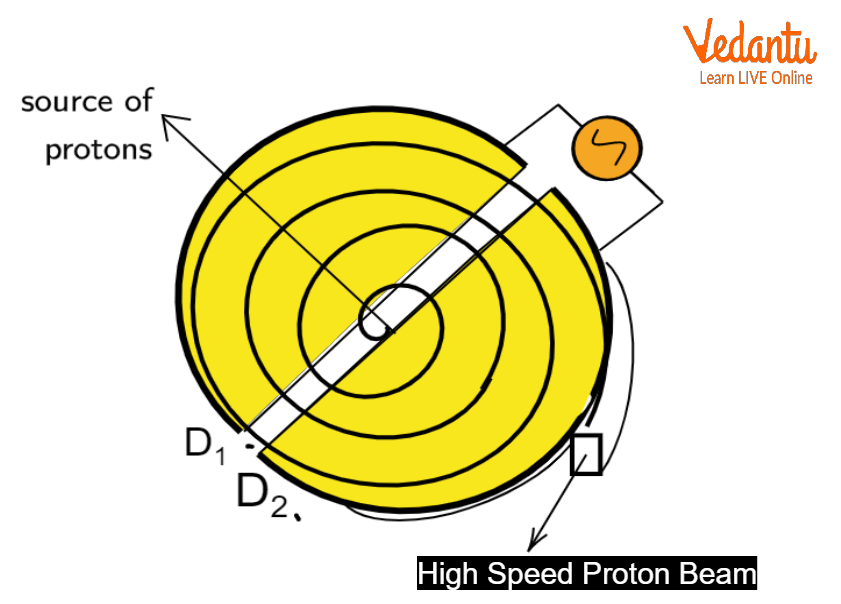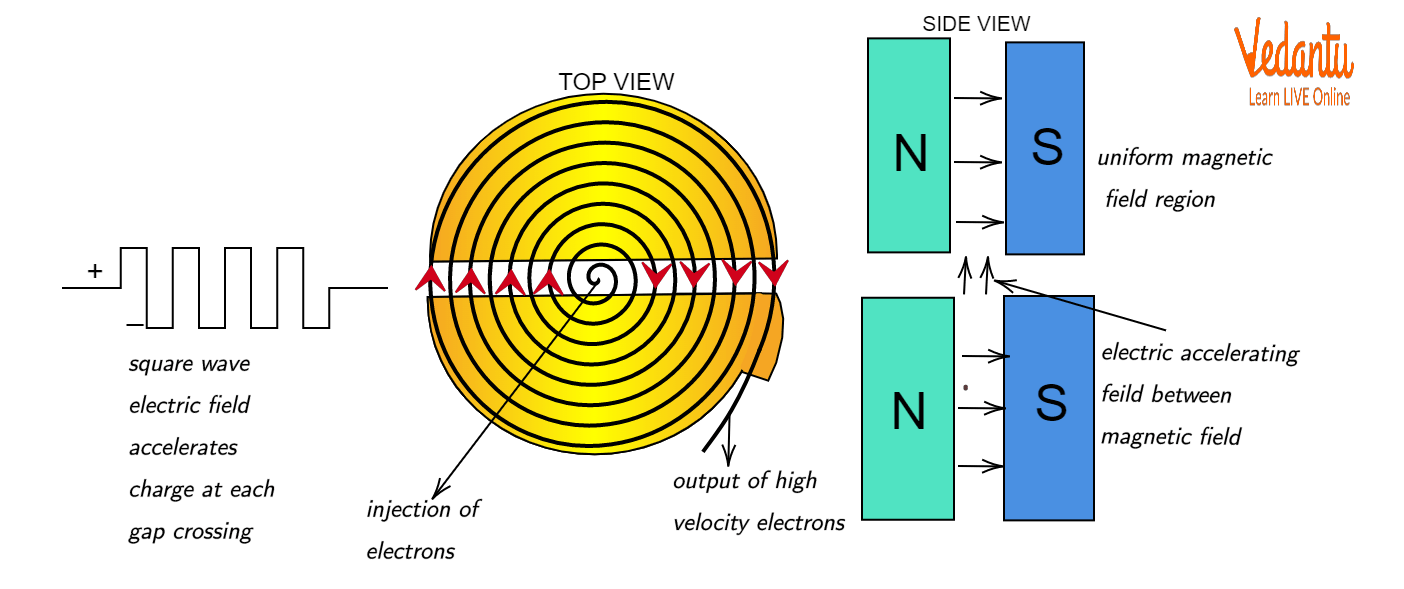Courses
Courses for Kids
Free study material
Free LIVE classes
More

# Cyclotron - JEE Important TopicLIVE
Join Vedantu’s FREE Mastercalss

## What is a Cyclotron?

It is a device used to accelerate charged particles like protons, deuterons, alpha-particles etc. To very high energies (velocities). It is also called a particle accelerator. Such high speed charged particles are used for

1. Studying nuclear structure/ Atomic structure

2. Inserting ions into solid structures

3. Study of Dual Nature

One method to accelerate charged particles to high speed/ Energy is using an electric field.

$F=qE$

Since, we know that acceleration is given as,

$a=\dfrac{F}{m}$

$\Rightarrow a=\dfrac{qF}{m}$

We know that

${{v}^{2}}={{u}^{2}}+2as$

$\Rightarrow {{v}^{2}}=\dfrac{2qEs}{m}$

$\Rightarrow v=\sqrt{\dfrac{2qEs}{m}}$

We can achieve high speed charged particles in the limited width of the electric field by making it pass through the limited Electric field a number of times.

## Principle of Cyclotron

Cyclotron is basically a device that is used to accelerate the charges (or positively charged particles) to relatively higher energies. The concept of Cyclotron was proposed by Lawrence.

Working Principle: The basic working principle of cyclotron is that a positively charged particle moving in a direction perpendicular to a magnetic field is influenced by magnetic Lorentz force as a result of which the particle starts moving in a circular path. The key points to be kept in mind are listed below.

1. A charged particle can be accelerated to very high speed/ energy by making it pass through a limited width of electric field a number of times.

2. This can be done with the help of a perpendicular Magnetic field (B) which rotates the charge a number of times in the field.

3. Perpendicular Magnetic field can rotate a charge in circles but cannot increase speed.

## Construction of Cyclotron

The cyclotron is constructed by dividing an evacuated chamber into two halves. These sections are named D1 and D2, also called “dees” due to their shapes. The arrangement of dees is done in such a manner that a very small gap is there between the dees. These dees are made to connect the terminal of an oscillator of high frequency having order of about 107 Hz and its potential is near about 10000 V. The evacuated Chamber containing the two dees is arranged in between the poles of an Electromagnet that is responsible for producing a strong magnetic field in a direction normal to the plane containing the dees. The positively charged particles originate from the gap present between the two dees. These charges are formed by ionization of the gas. Hydrogen, Deuterium and Helium are responsible for producing the protons, deuterons and the Alpha particles.Construction of a Cyclotron

## Working of Cyclotron

1. As positive charge emits from s, it is accelerated towards D1, Its speed increases.

2. When positive charge enters D1, the electric field is zero. Now Magnetic field acts on it, and charge moves in a circle with constant speed.Working of Cyclotron

1. As positive charge comes out of D1, polarity of Dees reverses, i.e. [D1-positive and D2-negative]

2. As positive charge enters D2, the electric field is zero and the perpendicular magnetic field rotates the charge in a circle of a larger radius.

3. As positive charge leaves D2, polarity of Dees reverses.

4. The positive charge accelerates towards D1, its speed further increases until it enters D.

In the end, the positive charge rotates in a very large circle, r = R (radius of the Dees). The charge q exits from the window through the deflector. The required centripetal force for keeping the particles in the curved path is:

${{F}_{C}}=\dfrac{m{{v}^{2}}}{r}$

The magnetic Lorentz force provides the required centripetal force as the particle moves in a circle of radius r and velocity v.

${{F}_{B}}=qvB$

$Bqv=\dfrac{\left( v{{m}^{2}} \right)}{r}$

$\dfrac{v}{r}=\dfrac{Bq}{m}=\text{constant}$       ….. (1)

Now, time taken to complete a semi-circle

$t=\dfrac{\pi r}{v}$                                                           ….. (2)

Now from equation (1) and (2),

$t=\dfrac{\pi m}{Bq}$                                                     ….. (3)

We can see from the from equation (3) the time taken to complete a semicircle is independent of following:

• Path

• Velocity(v) of particle

So, period of rotation T=2t

$T=\dfrac{2\pi m}{Bq}=\text{constant}$

The ion travels through each circle in precisely the same amount of time in a uniform magnetic field.

$v=\dfrac{1}{T}=\dfrac{Bq}{2\pi m}$           …… (5)

Resonance occurs if the high frequency oscillator is set to produce oscillations with the frequency specified in equation (5).

To accelerate protons, deuterons and $\alpha -$ particles.

$Bqv=\dfrac{m{{v}^{2}}}{r}$

Where $r=R$

So, $\left( R=\dfrac{mv}{qB} \right)$ .

Also $\dfrac{v}{r}=\dfrac{Bq}{m}=const$

$t=\dfrac{\pi m}{Bq}$

$t=\dfrac{\pi m}{Bq}$

$T=\dfrac{2\pi m}{Bq}=const$

$v=\dfrac{1}{T}=\dfrac{Bq}{2\pi m}$

## Applications of Cyclotron

Cyclotrons are observed to be better and more effective as compared to the linear accelerators. The reason is that the charged particles are accelerated by cyclotrons several times in the same setup and less space is required by cyclotrons as compared to linear accelerators, because of their cylindrical shapes. There are various applications of a cyclotron, few of which are listed below,

• One of the main purposes of cyclotrons is to accelerate positively charged particles in the experiments conducted in nuclear physics and these are also used to bombard atomic nuclei.

• These are also used for radiation therapy in cancer treatment.

• These can also be useful for nuclear transmutation, which means conversion of one chemical element or an isotope into another chemical element.

## Limitations of Cyclotron

Some of the limitations of a cyclotron are listed below.

1. Cyclotrons are not able to accelerate neutrons or neutral particles.

2. The cyclotrons cannot accelerate electrons. The reason is that the electrons are very small in size and lighter due to which the speed and their mass starts varying.

This can be explained on the basis of the following formula.

$m=\dfrac{{{m}_{0}}}{\sqrt{1-\dfrac{{{v}^{2}}}{{{c}^{2}}}}}$, where

m0 is the rest mass,

V is the velocity of the electron,

C is the speed of light

And m is the actual mass in motion.

1. Due to relativistic effects, the positively charged particles with relatively heavier mass cannot be accelerated by the Cyclotrons.

## Conclusion

• Cyclotron is a device that is used to accelerate charged particles. It is used for

1. Studying nuclear structure/ Atomic structure

2. Inserting ions into solid structures

3. Study of Dual Nature

• The principle of cyclotron is that when a charged particle is moving across a perpendicular magnetic field, it experiences magnetic Lorentz force and starts moving in a circular path.

• The applications of cyclotrons include radiation therapy for cancer treatment, nuclear transmutation, accelerating particles in nuclear experiments etc.

• Some of the limitations of a cyclotron are that it can’t accelerate neutral particles or electrons. It is also unable to accelerate heavier positively charged particles.

Last updated date: 02nd Oct 2023
Total views: 3.3k
Views today: 0.03k

## FAQs on Cyclotron - JEE Important Topic

1. What are the uses of Cyclotron?

The following are some used of cyclotron

• It is used in acceleration of charge particles during collisions and also used for other scientific purposes.

• It is used in Medical therapy for example like the method used in cancer tumors treatment with accelerated particles.

• It is used in the process of plasma being extracted from blood.

• It also used to speed up a particle in nuclear physics.

• Cyclotron has capacity of generating the radioisotopes which are used in medicines and imaging purposes.

2. Why do electrons, deuterons and protons accelerate by cyclotrons while neutrons not?

Protons: Protons are subatomic particles found in the atom's nucleus and have positive charge in it. Charged particles are accelerated by cyclotrons. Thus, cyclotrons accelerate protons.

Deuterons: Deuterons are hydrogen isotopes with a nucleus made up of one proton and one neutron. Since it has one proton, a particle with a positive charge is present in deuterons. So, deuterons are positively charged. So we can conclude that charged particles are accelerated by cyclotrons. Thus, cyclotrons accelerate protons, electrons and Deuterons.

Neutrons are subatomic particles that reside in the nucleus of an atom and have a mass equivalent to a proton. So neutrons are not charged. Thus, cyclotrons do not accelerate neutrons.